## Find a polynomial function of degree 7 with -2 as a zero of multiplicity​ 3, 0 as a zero of multiplicity3 ​, and 2 as a zero of multiplicity

Question

Find a polynomial function of degree 7 with -2 as a zero of multiplicity​ 3, 0 as a zero of multiplicity3 ​, and 2 as a zero of multiplicity 1.

in progress 0
2 months 2021-08-28T18:16:52+00:00 1 Answers 0 views 0

The polynomial is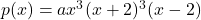, in which a is the leading coefficient.

Step-by-step explanation:

Zeros of a function:

Given a polynomial f(x), this polynomial has roots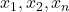such that it can be written as: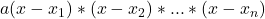, in which a is the leading coefficient.

-2 as a zero of multiplicity​ 3

This means that: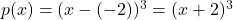0 as a zero of multiplicity 3

Then also: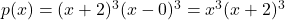2 as a zero of multiplicity 1.

Then: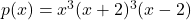The polynomial is, in which a is the leading coefficient.